Maharashtra State Board course SSC (English Medium) Class 9
Share

# The Monthly Maximum Temperature of a City is Given in Degree Celcius in the Following Data. - Algebra

ConceptFrequency Distribution Table

#### Question

The monthly maximum temperature of a city is given in degree celcius in the following data. By taking suitable classes, prepare the grouped frequency distribution table
29.2, 29.0, 28.1, 28.5, 32.9, 29.2, 34.2, 36.8, 32.0, 31.0, 30.5, 30.0, 33, 32.5, 35.5, 34.0, 32.9, 31.5, 30.3, 31.4, 30.3, 34.7, 35.0, 32.5, 33.5, 29.0, 29.5, 29.9, 33.2, 30.2
From the table, answer the following questions.
(i)  For how many days the maximum temperature was less than 34oC ?
(ii)  For how many days the maximum temperature was 34oC or more than 34oC ?

#### Solution

The monthly maximum temperature of a city is given in degree celcius in the following data:
29.2, 29.0, 28.1, 28.5, 32.9, 29.2, 34.2, 36.8, 32.0, 31.0, 30.5, 30.0, 33, 32.5, 35.5, 34.0, 32.9, 31.5, 30.3, 31.4, 30.3, 34.7, 35.0, 32.5, 33.5, 29.0, 29.5, 29.9, 33.2, 30.2
The grouped frequency distribution table of the given data is as follows:
 Class (Temperature) Tally marks Frequency 28-29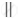2 29-30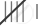6 30-31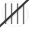5 31-32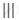3 32-33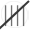5 33-34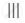3 34-35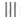3 35-36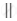2 36-37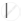1 Total N = 30
(i) The number of days the maximum temperature was less than 34oC = 2 + 6 + 5 + 3 + 5 + 3 = 24.
(ii) The number of days the maximum temperature was 34oC or more than 34oC = 3 + 2 + 1 = 6.
Is there an error in this question or solution?

#### APPEARS IN

Solution The Monthly Maximum Temperature of a City is Given in Degree Celcius in the Following Data. Concept: Frequency Distribution Table.
S